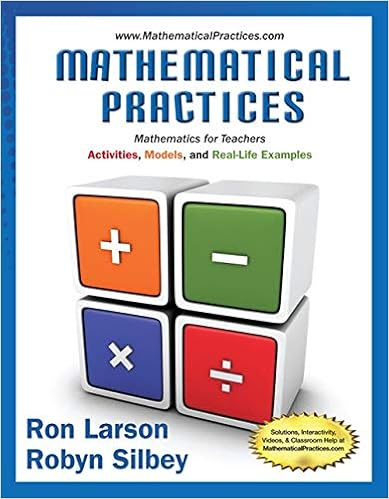# 84487 - Geometry Writing Assignment Introduction to...

• Homework Help
• 3
• 91% (23) 21 out of 23 people found this document helpful

This preview shows page 1 out of 3 pages.

##### We have textbook solutions for you!
The document you are viewing contains questions related to this textbook.The document you are viewing contains questions related to this textbook.
Chapter 10 / Exercise 31
Mathematical Practices, Mathematics for Teachers: Activities, Models, and Real-Life Examples
LarsonExpert VerifiedUnformatted text preview: Geometry Writing Assignment – Introduction to Triangles Each problem is worth 5 points Total Points: 50 Solve the problems pertaining triangles. Be sure to show all work when needed. 1. Explain your reasoning. 2 RW = RU . Kendra disagrees. Is either of them correct? 3 RU is bigger than RW so Jordan has to be wrong. Kendra is correct by disagreeing with him. a. Based on the figure, Jordan says that b. If QW = 27 inches, find QT. 27 is 2/3 of the total length, which means QT = 40.5 2. Jordan says that from the information supplied in the diagram, she can conclude that R is on the perpendicular bisector of QS . Robert disagrees. Is either of them correct? Explain your reasoning. Jordon is only partially correct. She can conclude that R is a bisector because of the lines that mark the congruency of the two halves of QS. There is no info that she can use to conclude that it is perpendicular. Jordon is wrong and Robert is correct by disagreeing with her. 3. Franklin measured and labeled the angles of a triangle as shown. Jennifer says that at least one of his measures is incorrect. Explain in at least two different ways how Jennifer knows that this is true. When you add up Franklin's angle measurements, you get a sum of 268 degrees. The sum of a triangles is always 180 degrees, which is not what Franklin labeled. That is how Jennifer knows that he is incorrent. 4. Joey says that triangle XYZ is obtuse. Robin disagrees because there are more acute angles then there are obtuse angles therefore it must be acute. Is either one of them correct? Explain your reasoning. Joey is correct because angle Z is greater than 90 degrees. An obtuse triangle is a triangle that has one obtuse angle and two acute angles, like the triangle in the picture. Robin is incorrect. 5. Compare and contrast the perpendicular bisectors and angle bisectors of a triangle. How are they alike? How are they different? Perpendicular bisectors and angle bisectors are similar because they divide sides and angles into equal parts. The main difference between them is that one divides a triangle's sides and the other divides the triangle's angles. Another difference is that the perpendicular bisector only creates right angles unlike the angle bisector. 6. Compare and contrast the perpendicular bisectors, medians, and altitudes of a triangle. How are they alike? How are they different? A median is a line segment that joins a vertex to the midpoint of the opposite side. The altitude is the perpendicular from the base to the opposite vertex of the triangle. Lastly, the perpendicular bisector is a perpendicular segment that passes through the midpoint and of a triangle. All three of them are similar in that they are all line segments that go to an opposite vertex. One of the differences is that the altitude is the only one of the three that must start at a base. 7a. Classify triangle ABC by its sides if side AB = 6x, side AC = 4x + 6 and side BC = 8x + 3. The perimeter of triangle ABC is 63. Show all your work. 6x + 4x + 6 + 8x + 3 = 63 , 18x + 9 = 63 , 18x = 54 , x = 3 AB = 6(3) = 18 , AC = 4(3) + 6 = 18 , BC = 8(3) + 3 = 27 , the triangle is an isosceles triangle. b. Explain your reasoning. Two of the sides are of equal length, so it is an isosceles triangle. 8. Is there enough information given to find the value of X. Explain your reasoning. There is not enough information to find the value of X. In order to find the value of X, I would need to know if the segments are congruent to each other by seeing the markings. Since there aren't any markings that tell me if they are congruent, I can't determine what the value of X is. ...
View Full Document

•••